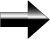The Order of Operations

This is the famous “PEMDAS” rule.  PEMDAS is an acronym that stands for “Parentheses, Exponents, Multiplication, Division, Addition, and Subtraction”.  It is the order of operations – which is to say that if we want to simplify an expression that has many different operation involved in it, PEMDAS is the order that we carry out the math in.

The very first thing we do is evaluate anything we can in that is inside the parentheses.  The next step is to evaluate the exponents.  After the exponents, we evaluate the multiplication and division.  Multiplication and division are equal in the eyes of PEMDAS – they must occur before addition and subtraction, and after parentheses and exponents, but it doesn’t matter if you multiply before you divide or if you divide before you multiply.  The final step is to perform all addition and subtraction.  Addition and subtraction are also on the same level as each other.  Addition and subtraction must be done last, but it doesn’t matter if add and then subtract or subtract and then add.

Let’s use the order of operations to do an example:

Simplify the expression: 3x(1+2)2

We have several different operations to carry out: there are parentheses, addition, multiplication, and an exponent.  The order we carry out the operations will affect the answer.  There are many different ways to carry out the operations, but is only one correct way to do it – using PEMDAS.

First, we will evaluate the terms inside the parentheses – we can add 1 to 2 and get 3.  Notice that even though we are adding, we are actually in the parentheses step of PEMDAS.  The addition step is within the parentheses.  If we had both multiplication and addition in the parentheses, we would first multiply, then add.  It’s like a PEMDAS within a PEMDAS.

We have: 3x(3)2

The next step is to evaluate the exponent.  32 = 3 * 3 = 9.  Another interesting point is that an exponent is a short-cut to multiplication.  33 = 3 * 3 * 3.  It makes sense for multiplication to come right after exponents.  Also, in the same way, multiplication is a short-cut for addition, and so multiplication comes before addition.

We now have: 3x * 9

The only thing left to do is perform the multiplication.  3 * 9 = 27, so our final simplified expression is now 27x

And so: 3x(1+2)2 = 27x

No matter how complicated an expression is, you can always simplify it by taking it one step at a time, and following PEMDAS, the order of operations.

Main Pre-Algebra Page